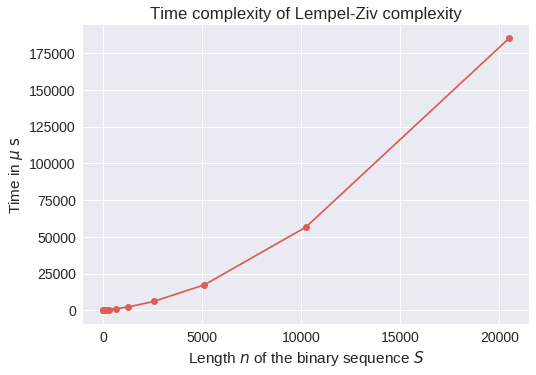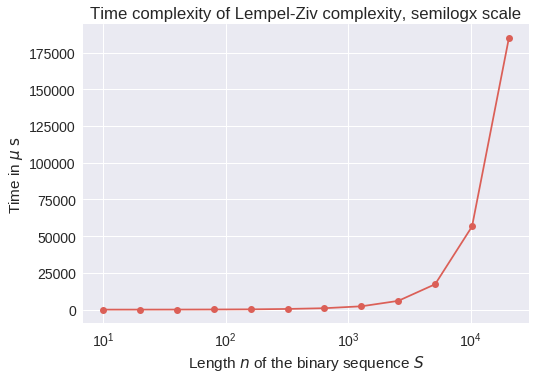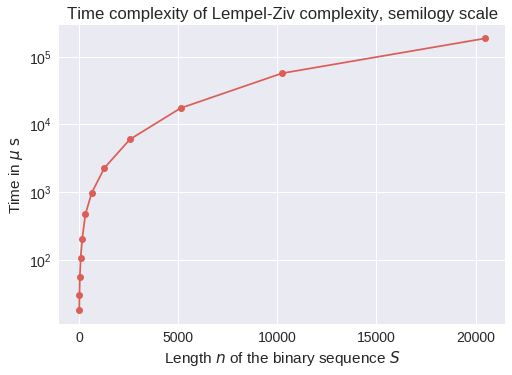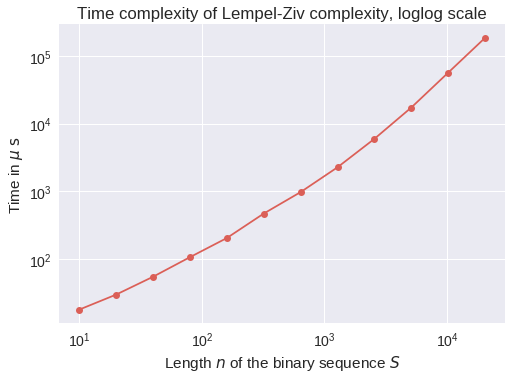# Short study of the Lempel-Ziv complexity¶

In this short Jupyter notebook aims at defining and explaining the Lempel-Ziv complexity.

I will give examples, and benchmarks of different implementations.

• Reference: Abraham Lempel and Jacob Ziv, « On the Complexity of Finite Sequences », IEEE Trans. on Information Theory, January 1976, p. 75–81, vol. 22, n°1.

## Short definition¶

The Lempel-Ziv complexity is defined as the number of different substrings encountered as the stream is viewed from begining to the end.

As an example:

>>> s = '1001111011000010'
>>> lempel_ziv_complexity(s)  # 1 / 0 / 01 / 1110 / 1100 / 0010
6


Marking in the different substrings, this sequence $s$ has complexity $\mathrm{Lempel}$-$\mathrm{Ziv}(s) = 6$ because $s = 1001111011000010 = 1 / 0 / 01 / 1110 / 1100 / 0010$.

Other examples:

>>> lempel_ziv_complexity('1010101010101010')  # 1 / 0 / 10
3
>>> lempel_ziv_complexity('1001111011000010000010')  # 1 / 0 / 01 / 1110 / 1100 / 0010 / 000 / 010
7
>>> lempel_ziv_complexity('100111101100001000001010')  # 1 / 0 / 01 / 1110 / 1100 / 0010 / 000 / 010 / 10
8


## Python implementation¶

In :
def lempel_ziv_complexity(binary_sequence):
"""Lempel-Ziv complexity for a binary sequence, in simple Python code."""
u, v, w = 0, 1, 1
v_max = 1
length = len(binary_sequence)
complexity = 1
while True:
if binary_sequence[u + v - 1] == binary_sequence[w + v - 1]:
v += 1
if w + v >= length:
complexity += 1
break
else:
if v > v_max:
v_max = v
u += 1
if u == w:
complexity += 1
w += v_max
if w > length:
break
else:
u = 0
v = 1
v_max = 1
else:
v = 1
return complexity


## Tests (1/2)¶

In :
s = '1001111011000010'
lempel_ziv_complexity(s)  # 1 / 0 / 01 / 1110 / 1100 / 0010

Out:
6
In :
%timeit lempel_ziv_complexity(s)

7.03 µs ± 457 ns per loop (mean ± std. dev. of 7 runs, 100000 loops each)

In :
lempel_ziv_complexity('1010101010101010')  # 1 / 0 / 10

Out:
3
In :
lempel_ziv_complexity('1001111011000010000010')  # 1 / 0 / 01 / 1110

Out:
7
In :
lempel_ziv_complexity('100111101100001000001010')  # 1 / 0 / 01 / 1110 / 1100 / 0010 / 000 / 010 / 10

Out:
8
In :
%timeit lempel_ziv_complexity('100111101100001000001010')

19.4 µs ± 2.31 µs per loop (mean ± std. dev. of 7 runs, 10000 loops each)


We can start to see that the time complexity of this function seems to grow exponentially as the complexity grows.

## Cython implementation¶

As this blog post explains it, we can easily try to use Cython in a notebook cell.

In :
%load_ext cython

In :
%%cython
from __future__ import division
import cython

ctypedef unsigned int DTYPE_t

@cython.boundscheck(False) # turn off bounds-checking for entire function, quicker but less safe
def lempel_ziv_complexity_cython(str binary_sequence):
"""Lempel-Ziv complexity for a binary sequence, in simple Cython code (C extension)."""
cdef DTYPE_t u = 0
cdef DTYPE_t v = 1
cdef DTYPE_t w = 1
cdef DTYPE_t v_max = 1
cdef DTYPE_t length = len(binary_sequence)
cdef DTYPE_t complexity = 1
# that was the only needed part, typing statically all the variables
while True:
if binary_sequence[u + v - 1] == binary_sequence[w + v - 1]:
v += 1
if w + v >= length:
complexity += 1
break
else:
if v > v_max:
v_max = v
u += 1
if u == w:
complexity += 1
w += v_max
if w > length:
break
else:
u = 0
v = 1
v_max = 1
else:
v = 1
return complexity


Let try it!

In :
s = '1001111011000010'
lempel_ziv_complexity_cython(s)  # 1 / 0 / 01 / 1110 / 1100 / 0010

Out:
6
In :
%timeit lempel_ziv_complexity_cython(s)

130 ns ± 8.72 ns per loop (mean ± std. dev. of 7 runs, 10000000 loops each)

In :
lempel_ziv_complexity_cython('1010101010101010')  # 1 / 0 / 10

Out:
3
In :
lempel_ziv_complexity_cython('1001111011000010000010')  # 1 / 0 / 01 / 1110

Out:
7
In :
lempel_ziv_complexity_cython('100111101100001000001010')  # 1 / 0 / 01 / 1110 / 1100 / 0010 / 000 / 010 / 10

Out:
8
In :
%timeit lempel_ziv_complexity_cython('100111101100001000001010')

259 ns ± 13.9 ns per loop (mean ± std. dev. of 7 runs, 1000000 loops each)


$\implies$ Yay! It seems faster indeed!

## Numba implementation¶

As this blog post explains it, we can also try to use Numba in a notebook cell.

In :
from numba import jit

In :
@jit("int32(boolean[:])")
def lempel_ziv_complexity_numba_x(binary_sequence):
"""Lempel-Ziv complexity for a binary sequence, in Python code using numba.jit() for automatic speedup (hopefully)."""
u, v, w = 0, 1, 1
v_max = 1
length = len(binary_sequence)
complexity = 1
while True:
if binary_sequence[u + v - 1] == binary_sequence[w + v - 1]:
v += 1
if w + v >= length:
complexity += 1
break
else:
if v > v_max:
v_max = v
u += 1
if u == w:
complexity += 1
w += v_max
if w > length:
break
else:
u = 0
v = 1
v_max = 1
else:
v = 1
return complexity

def str_to_numpy(s):
"""str to np.array of bool"""
return np.array([int(i) for i in s], dtype=np.bool)

def lempel_ziv_complexity_numba(s):
return lempel_ziv_complexity_numba_x(str_to_numpy(s))


Let try it!

In :
str_to_numpy(s)

Out:
array([ True, False, False,  True,  True,  True,  True, False,  True,
True, False, False, False, False,  True, False], dtype=bool)
In :
s = '1001111011000010'
lempel_ziv_complexity_numba(s)  # 1 / 0 / 01 / 1110 / 1100 / 0010

Out:
6
In :
%timeit lempel_ziv_complexity_numba(s)

6.16 µs ± 228 ns per loop (mean ± std. dev. of 7 runs, 100000 loops each)

In :
lempel_ziv_complexity_numba('1010101010101010')  # 1 / 0 / 10

Out:
3
In :
lempel_ziv_complexity_numba('1001111011000010000010')  # 1 / 0 / 01 / 1110

Out:
7
In :
lempel_ziv_complexity_numba('100111101100001000001010')  # 1 / 0 / 01 / 1110 / 1100 / 0010 / 000 / 010 / 10

Out:
8
In :
%timeit lempel_ziv_complexity_numba('100111101100001000001010')

9.35 µs ± 1.22 µs per loop (mean ± std. dev. of 7 runs, 100000 loops each)


$\implies$ Well... It doesn't seem that much faster from the naive Python code. We specified the signature when calling @numba.jit, and used the more appropriate data structure (string is probably the smaller, numpy array are probably faster). But even these tricks didn't help that much.

I tested, and without specifying the signature, the fastest approach is using string, compared to using lists or numpy arrays. Note that the @jit-powered function is compiled at runtime when first being called, so the signature used for the first call is determining the signature used by the compile function

## Tests (2/2)¶

To test more robustly, let us generate some (uniformly) random binary sequences.

In :
from numpy.random import binomial

def bernoulli(p, size=1):
"""One or more samples from a Bernoulli of probability p."""
return binomial(1, p, size)

In :
bernoulli(0.5, 20)

Out:
array([0, 0, 1, 0, 1, 1, 0, 1, 0, 0, 0, 0, 0, 0, 0, 0, 1, 1, 1, 1])

That's probably not optimal, but we can generate a string with:

In :
''.join(str(i) for i in bernoulli(0.5, 20))

Out:
'10011100011111111011'
In :
def random_binary_sequence(n, p=0.5):
"""Uniform random binary sequence of size n, with rate of 0/1 being p."""
return ''.join(str(i) for i in bernoulli(p, n))

In :
random_binary_sequence(50)
random_binary_sequence(50, p=0.1)
random_binary_sequence(50, p=0.25)
random_binary_sequence(50, p=0.5)
random_binary_sequence(50, p=0.75)
random_binary_sequence(50, p=0.9)

Out:
'11100011000111010111000110001110001110010010010010'
Out:
'00000100100000100000000000000000000100000000000000'
Out:
'00000010000010011100111010010000000000000010100010'
Out:
'11000111100001111001100000101011011011011010110110'
Out:
'11111001110101111111111101111111010111111111110111'
Out:
'11111111111111101111101111110111111001111110111111'

And so, this function can test to check that the three implementations (naive, Cython-powered, Numba-powered) always give the same result.

In :
def tests_3_functions(n, p=0.5, debug=True):
s = random_binary_sequence(n, p=p)
c1 = lempel_ziv_complexity(s)
if debug:
print("Sequence s = {} ==> complexity C = {}".format(s, c1))
c2 = lempel_ziv_complexity_cython(s)
c3 = lempel_ziv_complexity_numba(s)
assert c1 == c2 == c3, "Error: the sequence {} gave different values of the Lempel-Ziv complexity from 3 functions ({}, {}, {})...".format(s, c1, c2, c3)
return c1

In :
tests_3_functions(5)

Sequence s = 11010 ==> complexity C = 3

Out:
3
In :
tests_3_functions(20)

Sequence s = 00011000000010010111 ==> complexity C = 6

Out:
6
In :
tests_3_functions(50)

Sequence s = 00111111110000010000100011000010001100110001001101 ==> complexity C = 8

Out:
8
In :
tests_3_functions(500)

Sequence s = 01011110110110010010101101110000101110100011011101100101001000001100111000011000101000000010010010010100101101101010111010001100011000100111111101101111000010001111001110010000001100011011111001001011101110001000111101100110100111011010101000010100011000010001101101000101111101001011111001100111001010100010010001111000101100110000100000010100111111000110110011110101000100110101000011001010111010101111101100111010101011110001100001111111000100100000001000100100101011010111011001101110110111111110 ==> complexity C = 60

Out:
60
In :
tests_3_functions(5000)

Sequence s = 00001100000110000110100011111111010111110000010110100111111000000111011011000001011101101101011100101110010001010000011011110100001011001011000101100001000000110100110011111100010111000101000011100010010100011110011110011101011101110011110001110110100100111110010010110010100100100101001010110100111111000010011110101011110001010010000110101000000111001010101000101001101000111100001100101111010010001011111111110001111001100011010011101011001111110110011101100011001000001000110101001010000000101011111101010110111110000000001000111000000011110101001110101011111100000101011111000100000000110110011011011111001111101000110001011111011010011100100110010001011011001001100111000001100111101011000010100010111100010000111001111001100001010001010010100110101111001010011010110001100001100011110011101101000110000010010010111110101011000000101110110011100110110011111111010011001101011101010001110001000001000000001111010000000010000101011001111001101110100110000100110000010010011110111001001111110100101000010101001101011111111010010110011000011001101001110010100010111100001011001000011000001000011111001000011110001010111111111100000000110101110110001100010111100101100010001001101010101000110010100101010100001111010001001001111010100010101101101110010110011001010110101111010011000100011110001110011000001000010010000000000001000001011010100010111001001000101000001101100100011100011110011010010010110011000101110011110000010000100110100100000011011100110111101110110101110010111000011110100110001011010111000111001110101110001100000111011100011100000010000101010110010011000000001111100101010101110100111111101011001100101111000011101111010101001000111001110100100011110000110001110100010101001100101010000101101000010110010000011100000111001111101110100010011010111101000010100011011100111001110000001000011110111001110100110010110000010011111101000011101010000001011101011111100110111010111000101110010011110110110000000110110000110010000011110000111000101001001101011101101101010011010100101000011101010110011100000110100010001101110101001011111110100101010011111110100111000110010111010100000100101001010100000000000000101001111111001010010111011000000001100111111011011100100011011100010101000111000000100101100000110111100101100100110010001000000111000010011100001010111010001011111111011101010101101101000100010110101110001110100101001111101111101100110100101100110111001011011110011110100010100010110101110110000111000100000010101011100101010101100001011100101000100110100110010100101100010010010100010100110001010101001011001100001001111110000101000000111110001101010110001000000111111111101100001101011011000110000010111111101111010001100111011100111001100010110111010110011011100111101110010100011011011000001011111111001000001010001010001100011010101011100010000101111111101000100110110110001110111100011011101010100101010100111011111111001001000000001010001100100111111000111010110111100101001101100001100101101000001111010110000010101000010101000110110001100101010110111110111110110110101101000010101000001111101100001100010111111111001101111000111110100111001010111111110000111111100100110010000101011111010100001001101010101010111111110100010010010101000100011100101011101100001010011100011101111111100000100100111101011011000110010011111110000011010011000111110110110110000111010101101100101101100010100101011010001110111011111100010011011100110000000011111011010001101110110000110001101011111110011011110001111100111011001100011111010010000111000000110001101010101011010000110110011111010101111101001100000000110001111010110110001111111010100001100101000011011101010111000010110110010100001010011010010101011011100001010101011100111011110001100110101000011001000010100111000100110001000110111100010100110111011111101110011000100000011100010011001010001110010011011111110111100000111001010110000011011010011010011000000111100011101101110110101010010001111100100011100001101011001010101001100101101101011010011001011001100100011010001011000101011000011000101010110000110000011111111101000001100011100111110110001000111000111111011111110101000001100001100010111001101011100001010011000001110100010011001001101101111010100100001010011110100110001000001010010110111110001011101000000110011111001110011011011100111000101010101010011100100001000011001100001101100011100110101010010011110101110000111000001111010100011111100011101000010101111010001101100010111111110110000101010111000000101111001001111110011110001110111101000010010001101000001011100010000011000000011101010100000101001001100101001001001001001111111101100111001110110001000001100001010011001001110111111101111100011100011101001000000100000010111101001101001100010101001110100101000010110101011100011111100001100110111010111111110010001000011000101110111110010100011111111001001011110010101111101110111101000000001111010000010001100011011010001101110010110111011000110110111100111111011001000101101011000101110010011010010111001000101000111010101000000010111111010011001110010110001010001111011110001101001100000110000001 ==> complexity C = 420

Out:
420

## Benchmarks¶

On two example of strings (binary sequences), we can compare our three implementation.

In :
%timeit lempel_ziv_complexity('100111101100001000001010')
%timeit lempel_ziv_complexity_cython('100111101100001000001010')
%timeit lempel_ziv_complexity_numba('100111101100001000001010')

16.7 µs ± 471 ns per loop (mean ± std. dev. of 7 runs, 100000 loops each)
249 ns ± 6.71 ns per loop (mean ± std. dev. of 7 runs, 1000000 loops each)
7.98 µs ± 236 ns per loop (mean ± std. dev. of 7 runs, 100000 loops each)

In :
%timeit lempel_ziv_complexity('10011110110000100000101000100100101010010111111011001111111110101001010110101010')
%timeit lempel_ziv_complexity_cython('10011110110000100000101000100100101010010111111011001111111110101001010110101010')
%timeit lempel_ziv_complexity_numba('10011110110000100000101000100100101010010111111011001111111110101001010110101010')

139 µs ± 4.33 µs per loop (mean ± std. dev. of 7 runs, 10000 loops each)
1.94 µs ± 83.4 ns per loop (mean ± std. dev. of 7 runs, 1000000 loops each)
22 µs ± 364 ns per loop (mean ± std. dev. of 7 runs, 10000 loops each)


Let check the time used by all the three functions, for longer and longer sequences:

In :
%timeit tests_3_functions(10, debug=False)
%timeit tests_3_functions(20, debug=False)
%timeit tests_3_functions(40, debug=False)
%timeit tests_3_functions(80, debug=False)
%timeit tests_3_functions(160, debug=False)
%timeit tests_3_functions(320, debug=False)

28.2 µs ± 2 µs per loop (mean ± std. dev. of 7 runs, 10000 loops each)
52.3 µs ± 2.75 µs per loop (mean ± std. dev. of 7 runs, 10000 loops each)
108 µs ± 1.42 µs per loop (mean ± std. dev. of 7 runs, 10000 loops each)
359 µs ± 91.7 µs per loop (mean ± std. dev. of 7 runs, 1000 loops each)
862 µs ± 64.2 µs per loop (mean ± std. dev. of 7 runs, 1000 loops each)
3.16 ms ± 299 µs per loop (mean ± std. dev. of 7 runs, 100 loops each)

In :
def test_cython(n):
s = random_binary_sequence(n)
c = lempel_ziv_complexity_cython(s)
return c

In :
%timeit test_cython(10)
%timeit test_cython(20)
%timeit test_cython(40)
%timeit test_cython(80)
%timeit test_cython(160)
%timeit test_cython(320)

17.1 µs ± 490 ns per loop (mean ± std. dev. of 7 runs, 10000 loops each)
28.8 µs ± 959 ns per loop (mean ± std. dev. of 7 runs, 10000 loops each)
62.4 µs ± 6.77 µs per loop (mean ± std. dev. of 7 runs, 10000 loops each)
125 µs ± 7.15 µs per loop (mean ± std. dev. of 7 runs, 10000 loops each)
216 µs ± 13.8 µs per loop (mean ± std. dev. of 7 runs, 1000 loops each)
459 µs ± 16.7 µs per loop (mean ± std. dev. of 7 runs, 1000 loops each)

In :
%timeit test_cython(640)
%timeit test_cython(1280)
%timeit test_cython(2560)
%timeit test_cython(5120)

1.18 ms ± 214 µs per loop (mean ± std. dev. of 7 runs, 1000 loops each)
2.38 ms ± 144 µs per loop (mean ± std. dev. of 7 runs, 100 loops each)
5.93 ms ± 71.1 µs per loop (mean ± std. dev. of 7 runs, 100 loops each)
17.1 ms ± 168 µs per loop (mean ± std. dev. of 7 runs, 100 loops each)

In :
%timeit test_cython(10240)
%timeit test_cython(20480)

52.1 ms ± 584 µs per loop (mean ± std. dev. of 7 runs, 10 loops each)
178 ms ± 6.03 ms per loop (mean ± std. dev. of 7 runs, 10 loops each)


## Complexity ?¶

$\implies$ The function lempel_ziv_complexity_cython seems to be indeed (almost) linear in $n$, the length of the binary sequence $S$.

But let check more precisely, as it could also have a complexity of $\mathcal{O}(n \log n)$.

In :
import matplotlib.pyplot as plt
import seaborn as sns
%matplotlib inline
sns.set(context="notebook", style="darkgrid", palette="hls", font="sans-serif", font_scale=1.4)


It's durty, but let us capture manually the times given by the experiments above.

In :
x = [10, 20, 40, 80, 160, 320, 640, 1280, 2560, 5120, 10240, 20480]
y = [18, 30, 55, 107, 205, 471, 977, 2270, 5970, 17300, 56600, 185000]

In :
plt.figure()
plt.plot(x, y, 'o-')
plt.xlabel("Length $n$ of the binary sequence $S$")
plt.ylabel(r"Time in $\mu\;\mathrm{s}$")
plt.title("Time complexity of Lempel-Ziv complexity")
plt.show()

Out:
<matplotlib.figure.Figure at 0x7f54a1380f60>
Out:
[<matplotlib.lines.Line2D at 0x7f54a0e2b550>]
Out:
<matplotlib.text.Text at 0x7f54a0ec7240>
Out:
<matplotlib.text.Text at 0x7f54a0e5f0b8>
Out:
<matplotlib.text.Text at 0x7f54a0e82f60>In :
plt.figure()
plt.semilogx(x, y, 'o-')
plt.xlabel("Length $n$ of the binary sequence $S$")
plt.ylabel(r"Time in $\mu\;\mathrm{s}$")
plt.title("Time complexity of Lempel-Ziv complexity, semilogx scale")
plt.show()

Out:
<matplotlib.figure.Figure at 0x7f549d598400>
Out:
[<matplotlib.lines.Line2D at 0x7f549d539470>]
Out:
<matplotlib.text.Text at 0x7f549d5741d0>
Out:
<matplotlib.text.Text at 0x7f54a0dfc860>
Out:
<matplotlib.text.Text at 0x7f549d54a358>In :
plt.figure()
plt.semilogy(x, y, 'o-')
plt.xlabel("Length $n$ of the binary sequence $S$")
plt.ylabel(r"Time in $\mu\;\mathrm{s}$")
plt.title("Time complexity of Lempel-Ziv complexity, semilogy scale")
plt.show()

Out:
<matplotlib.figure.Figure at 0x7f549d4b7198>
Out:
[<matplotlib.lines.Line2D at 0x7f549d552470>]
Out:
<matplotlib.text.Text at 0x7f549d4cf240>
Out:
<matplotlib.text.Text at 0x7f549d4ab160>
Out:
<matplotlib.text.Text at 0x7f549d552278>In :
plt.figure()
plt.loglog(x, y, 'o-')
plt.xlabel("Length $n$ of the binary sequence $S$")
plt.ylabel(r"Time in $\mu\;\mathrm{s}$")
plt.title("Time complexity of Lempel-Ziv complexity, loglog scale")
plt.show()

Out:
<matplotlib.figure.Figure at 0x7f549d53f358>
Out:
[<matplotlib.lines.Line2D at 0x7f549d29bcf8>]
Out:
<matplotlib.text.Text at 0x7f549d326e10>
Out:
<matplotlib.text.Text at 0x7f549d3180b8>
Out:
<matplotlib.text.Text at 0x7f549d2abac8>It is linear in $\log\log$ scale, so indeed the algorithm seems to have a linear complexity.

To sum-up, for a sequence $S$ of length $n$, it takes $\mathcal{O}(n)$ basic operations to compute its Lempel-Ziv complexity $\mathrm{Lempel}-\mathrm{Ziv}(S)$.

## Conclusion¶

• The Lempel-Ziv complexity is not too hard to implement, and it indeed represents a certain complexity of a binary sequence, capturing the regularity and reproducibility of the sequence.

• Using the Cython was quite useful to have a $\simeq \times 100$ speed up on our manual naive implementation !

• The algorithm is not easy to analyze, we have a trivial $\mathcal{O}(n^2)$ bound but experiments showed it is more likely to be $\mathcal{O}(n \log n)$ in the worst case, and $\mathcal{O}(n)$ in practice for "not too complicated sequences" (or in average, for random sequences).

Thanks for reading! My implementation is now open-source and available on GitHub, on https://github.com/Naereen/Lempel-Ziv_Complexity.

It will be available from PyPi very soon, see https://pypi.python.org/pypi/lempel_ziv_complexity.

See this repo on GitHub for more notebooks, or on nbviewer.jupyter.org.

That's it for this demo! See you, folks!